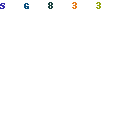ON1DHT's 50 MHz converter50 MHz converter technical specifications

• Frequency range from 50.000 MHz to 52.000 MHz
• In 50 Mc out 28 Mc
• Power supply = 11...18v max
• Total power consumption = 15mA (LED included)
• Power consumption IC1 = 2,5 mA
• Sensitivity = 0.22uV at 12dB SINAD
• Mixer noise figure = 4,6dB
• Input impedance = 50 Ohm
• Output impedance = 50...600 Ohm
• Local oscillator 22MHz
• Frequency stability = +-10Hz
• Operating ambient temperature range = -40 to +85°C

SCHEMATICPARTS

IC1 = NE602 or SA602
IC2 = 78L06
T1 = BC547
C1, C4 = 10uF/25v
C2, C3 = 100nF
C5 = 47uF/16v (tantaal)
C6, C15 = 47nF (polyester)
C7 = 47pF
C8 = 22pF
C9 = 0...22pF (green)
C10 = 2n2
C11= 10nF
C12 = 0...40pF (white)
C13 = 47pF
C14 = 39pF
C16,C17 = 330pF
C18 = 100pF
C19 = 22nF
C20, C21 = 470pF
P1 = 100 Ohm
R1 = 1k
R2 = 2k2
R3 = 100 Ohm
R4 = 5k6
R5 = 1k
P1 = 100 Ohm

Coil specifications:
L1 = 7 wnd 1mm silver 9mm coildiameter (drill 7), tap on 1,5 wnd from the cold end.
L2 = 11 wnd 0,6mm email 5mm coildiameter (drill 4)
L3, L4 = shokes (RFC) 10uH

Notes:
Tune to the desired bandpass frequency (50Mc) with C9 until you have the best reception.
Use C12 to calibrate the output frequency to your receiver. The output frequency can be adjusted up to 300Hz.
The output HF-level can be adjusted with P1. Regulate it according to the sensitivity of your receiver.

Other output frequencies can be set by changing the 22MHz Xtal: Example: output frequency is 26 MHz then you use a 24 MHz Xtal (50MHz - 24MHz = 26MHz).
Build the converter in a metal box and use small connections between the parts.

Important: use only a antenna designed for 50MHz! A simple dipole of around 3 meters in length (two times 1,45 meters) will work just fine if the propagation is there. Look at my homebrew site for a 3-element beam that works much better then a dipole and gives more gain, or a my 5/8 lambda vertical.

PCB:
A PCB has been designed for this project by ON1MFW. E-mail me for detailed PCB. PCB-parts side PCB layout

Detailed information and manual (in Flemish (Nederlands)) ON1DHT's 50MC CONCVERTER MANUAL

Technical graphs:This project has been published in CQ-QSO (in Dutch and French) the ham-radio magazine of the UBA.
Magzine 06-07/2000 pages 14,15,16 and 17.